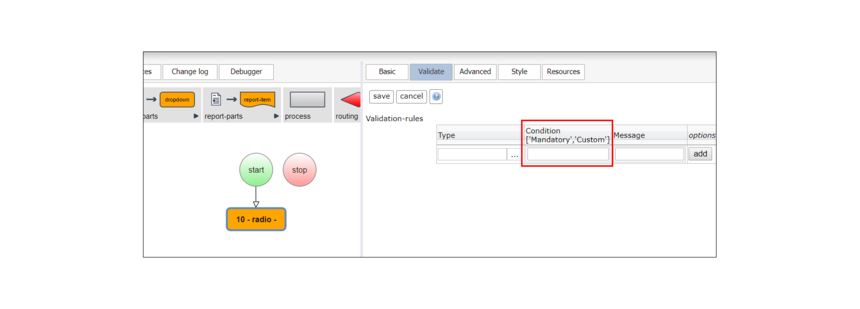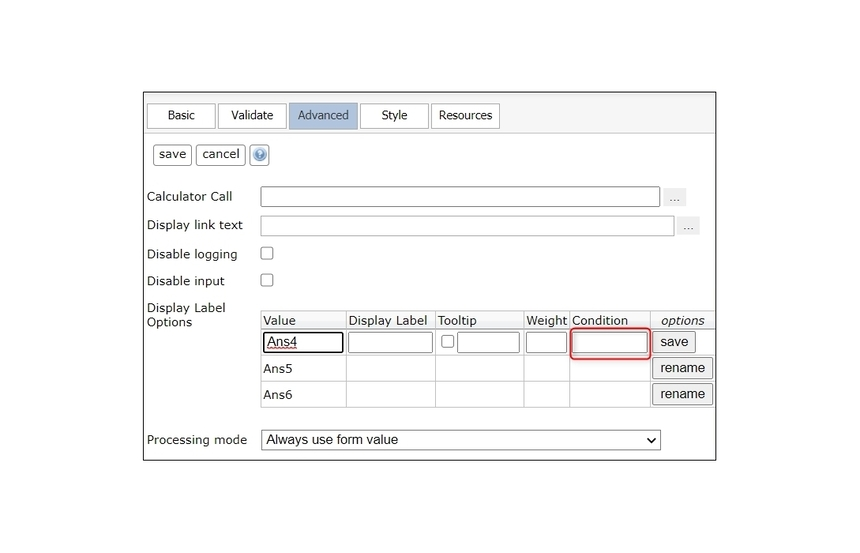0

# Setting Conditions (Data Validation)

## Description

The checklist and radio list form parts allow you to set conditions the system verifies before displaying an option or validating a user's selection. It is important to use the right syntax in these Condition fields, however, so the system knows what you want it to validate.

Node Type:  Condition is a field in Editor of the radio list and checklist form part nodes. It is a field found in most nodes on the Validate tab.

Used With: Condition syntax is used with nodes in all frameworks.

## Part Editor

The following images show you where to find the Condition field in radio lists and checklists.

At the question level, validation is found on the Validate tab:For each item, conditions can be specified in the Advanced tab:## Using Syntax

The most important tip in using the Condition field in a node is to use the correct syntax, and the data object type - are you using text or a number - is the important factor when determining the syntax you should use in the Condition field. If you are comparing text you want to compare against text and if you are comparing a number you want to compare against another number. For example:

\$(number_variable)==0
'\$(text_variable)'=='value' (note that text objects/values are in single parentheses)

#### Operators

In the examples above, the == is an operator. This is the symbol that tells the system what you want to do. In the Condition field you use operators and additional parentheses to set up simple and complex rules. LogicNets recognizes the following operators in the Condition field:

==          equals
~=          does not equal
> or >=   greater than, greater than or equal to (numeric only)
< or <=   less than, less than or equal to (numeric only)

Note: "Contains" is not supported until version 7.3 (see below). When setting up conditions on a collection, use the collection_object construction.

#### Examples of the Correct Syntax

Text Objects

('\$(cancer_risk)'=='High Risk' or '\$(signs_infection)'=='yes')
('\$(cancer_risk)'=='High Risk' and '\$(signs_infection)'=='no')
('\$(cancer_risk)'=='Low Risk' and '\$(signs_infection)'=='yes' and '\$(risk_infection)'=='moderate')

Numeric Objects

\$(test_score)==0
\$(test_score)==1
\$(test_score)>=2

#### Pattern Matching Validation

You can also use validation to match simple or complex patterns. For more information on pattern matching, visit https://www.lua.org/pil/20.2.html

Examples:

A             The strfind below, when pasted into the condition box, will match the input for any alphanumeric in the format AA-AAAAAAA.

``strfind('\$(variable-name)','^[%d,%u][%d, %u]%-[%d, %u][%d, %u][%d, %u][%d, %u][%d, %u][%d, %u][%d, %u]\$')``

B             The following example matches for date formats:

``strfind('\$(date_variable)','^[0-9].[0-9].%d%d%d%d\$')``

#### Using Collection Variables

You can use collection variables to have the system display an answer option in a checklist if another variable contains a particular word or phrase; for example, "ABC". By using an XPath query, you can write conditions that verify whether a collection contains specific answers. To do this, enter in the condition field a condition using the following syntax:   \$(? [xpath query]) ~=' '   (Use single quotes with no space between them.)

As an example, if you enter in the condition field the query below the system will only display the answer option only IF the data collection called "some_variable" contains A as one of its answers.

``\$(?/some_array/[contains(this, 'A')]) ~= ' '  or this will also work:    ``
``\$(?/some_array[_.this == 'A']) ~=' '``
``The above can be expanded for more complex validations and included with other text and numeric validations as follows:``

``\$(?/some_array/[contains(this, 'A') or contains(this, 'B')]) ~= ' '  ``
``   or this will also work:    \$(?/some_array[_.this=='A' or _.this=='B']) ~=' '``

You can also use OR and AND and parentheses to make your validation more complex:

``\$(?/some_array[_.this == 'A']) ~=' ' or '\$(text_variable)'=='value' ``
``\$(?/some_array[_.this == 'A']) ~=' ' and (\$(number_variable)==0 or '\$(text_variable)'=='value')``

#### "Does Not Include"

To instruct the system to include an answer option only when another variable DOES NOT INCLUDE a value is more complex, because that variable may or may not exist. For simple collections (like checklist objects), you can do this by having the system verify how many "hits" the XPath query generates. The syntax to use for DOES NOT INCLUDE is '\$(? [xpath query])' ~= '1'.

For example, if you use the condition below for an answer option the system will only display the answer option IF the data collection called "some_array" does NOT contain A as one of its answers.

``'\$(?/some_array/[contains(this, 'A')])' ~= '1'``

In the example above, using the ' ' will convert the XPath output to a number of hits or the count of objects that include the value 'A'. The output is represented as text, so any comparisons must also be in single quotes.

• {"A", "A","B"} will return '1' as there is only one object with 'A' (even though 'A' appears multiple times)

• {"AA", "B", "C" } will return '0', as there is no object with 'A' ('AA' will not trigger a match)  [Note that  '0' ~= '1' is TRUE, and therefore the answer option WILL display]

#### Simple Approach

In large or complex collections, where the XPath query has to be defined more tightly to isolate only the target element of the collection, it is always possible to define rules and internal data objects prior to a form part node, rather than in the Conditions field, and use those interim data objects in simpler validation rules.

It is good practice to develop these rules longhand and verify all eventualities are covered as part of the development process. We recommend using this approach for any validation of arrays that cannot be handled by the simple examples above.

## Example